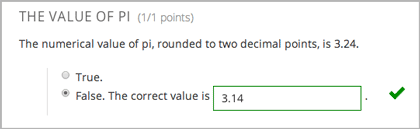# 10.26. Multiple Choice and Numerical Input Problem¶

Note

EdX does not support this problem type.

You can create a problem that combines a multiple choice and numerical input problems. Students not only select a response from options that you provide, but also provide more specific information, if necessary.Note

Currently, students can only enter numerals in the text field. Students cannot enter words or mathematical expressions, which might be confusing to students who are accustomed to other edX numerical input fields.

You can make a calculator available to your learners on every unit page. For more information, see Calculator Tool.

## 10.26.1. Create a Multiple Choice and Numerical Input Problem¶

To create a multiple choice and numerical input problem, follow these steps.

1. In the unit where you want to create the problem, click Problem under Add New Component, and then click the Advanced tab.
3. In the component that appears, click Edit.
4. In the component editor, paste the code from below.
5. Replace the example problem and response options with your own text.
6. Click Save.

## 10.26.2. Multiple Choice and Numerical Input Problem Code¶

<problem>
The numerical value of pi, rounded to two decimal places, is 3.24.
<choicetextresponse>